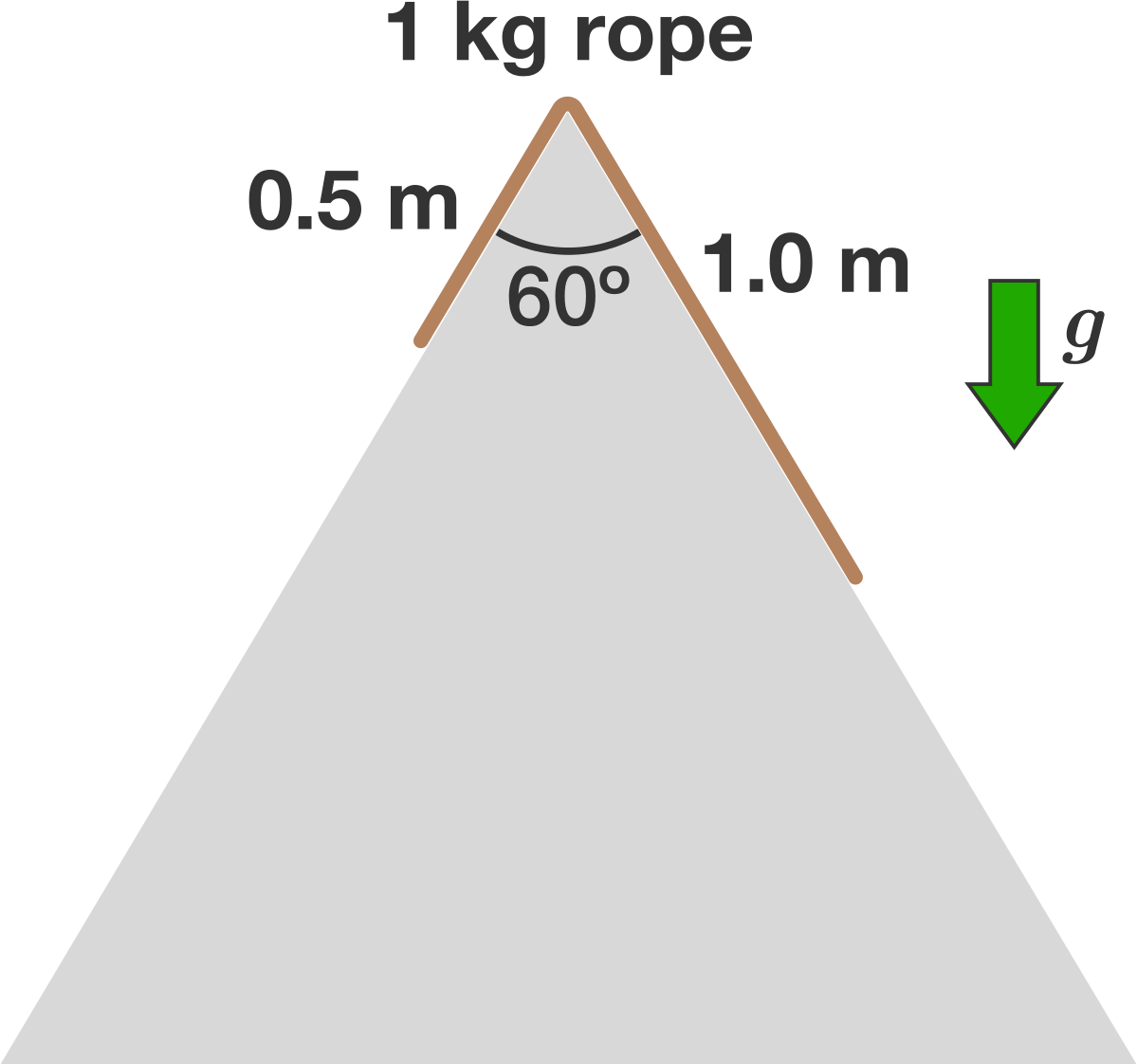If a five-hour candle and a six-hour candle were lit at the same time, how much time would elapse before one............

+1 vote
73 views

If a five-hour candle and a six-hour candle were lit at the same time, how much time would elapse before one candle would be five times longer than the other?posted Aug 17

L - length of the candle
x = hours spent on candle burning.
5L((5 - x)/5) = L((6 - x)/6)
5*(5 - x)*6 = 5*(6 - x)
150 - 30x = 30 - 5x
25x = 120
x = 120/25 = 4 hours & 48 minutes.answer Aug 18

Similar Puzzles

The ratio between the rates of walking of A and B is 2:3 and therefore A takes 10 minute more than the time taken by B to reach the destination.

If A had walked at double the speed, how much time he would have taken to reach the destination.

A 1kg rope of length 1.5m slides down a frictionless double ramp in the shape of an equilateral triangle, as shown in the image below. At time t=0, the rope is at rest with of its length 1m on the right side of the ramp.

How much time elapses before all of the rope is on the right side?

Note: Assume g=10Before a final time elapse
There will have been at least two gaps

During gaps, folks time they're biding
While a dozen halt their sliding

When a certain red line is crossed
It means that you may have just lost

A masked man sometimes saves the day
Stopping projectiles aimed his way

The appearance of a red light
Brings both vexation and delight

What am I?